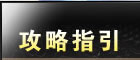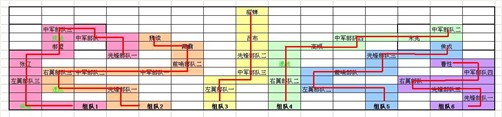• 服务时间：7X24小时专业服务
• 客服热线：400-082-6660
• 客服邮箱：gm@niua.com看到《4分30秒完成虎牢关》的帖子，觉得这篇攻略仍然不够完美，作者没有考虑到道具的提高，作战线路也有更优的方式，因此有人在4分30之前就完成了。所以本人也来写一篇吕布虎牢关的攻略，想要挑战一下最快完成虎牢关的时间。

由于挑战的是最快完成的速度，故对组队成员有一下几点要求。

1、组员必须6个全满

2、要求组员能够不用补给打完地图

3、网络要求快，不能有延时

另外对战役地图说明如下：

1、攻击一只部队等待时间为30秒

2、移动一步等待时间15秒

3、道具中只有速战和疾驰能提高时间，使用道具有效时间均为2分钟，道具效果无法累加，使用速战后攻击一直部队等待时间减半，即15秒，使用疾驰后移动一步等待时间减半。

4、疾驰在得到后立即使用，速战在攻击下一个部队前使用。

针对战役地图敌方部队、道具、出战位置均固定，本人设计了最佳的作战路线如下：为了计算方便，将移动cd计为1,攻击cd计为2,使用速战和疾驰后，移动和攻击cd分别为0.5和1.

组队1=1+0.5×3+2+0.5+2+0.5×2+2+0.5+2+0.5+2+0.5×2=16

组队2=1+1+2+12=16

组队3=1+2+1×2+2+1+2+1+2+1×2=15

组队4=1×2+2+10=14

组队5=1×3+2+1×2+2+1×2+2+1×2=15

组队6=1+2+1+2+1+2+1×2+2+1=14

（最后的侯成4、5、6组均可以完成，速度快者优先）

由上可以计算出完成战役的时间为16×15=240秒=4分。

最后，这次新版的战役地图设计得非常不错，既要求组员有很高的个人实力，又需要很好的团队配合，但是固定的敌方部队、道具和出站位置，设计的非常失败，大大降低了地图的可玩性，玩过几遍研究透彻之后，打法就变得单一和程式化，希望能在下次更新版本的时候有所修改。另外建议傲视能对战役地图最佳记录的完成者有特殊奖励，以提高玩战役地图的积极性。

牛A《傲视天地》官方网站：http://astd.niua.com/
最牛的游戏尽在牛A平台！http://www.niua.com/